# DAX实现K近邻(KNN)分类算法

## 一、K近邻算法简介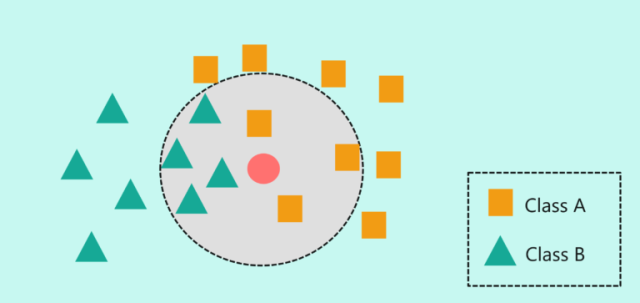## 二、利用DAX的实现过程

#### 1.准备工作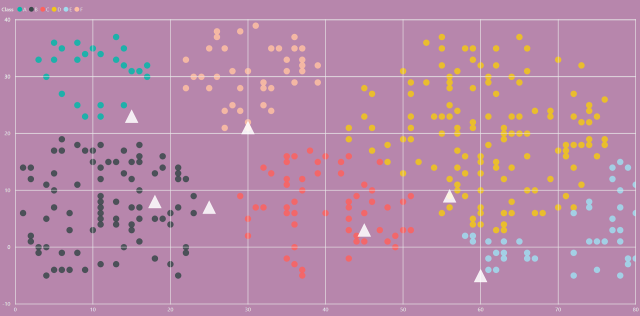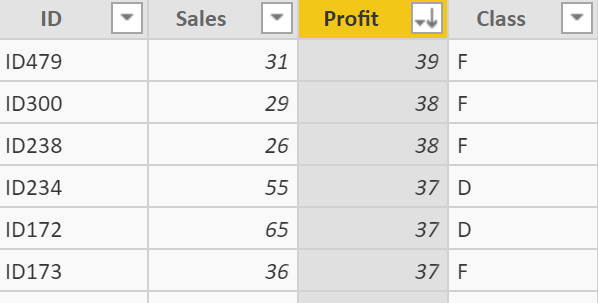``````测试集 = DataTable("_ID", STRING,
"_Sales", INTEGER,
"_Profit",INTEGER
,{
{" ID501",15,23},
{" ID502",25,7},
{" ID503",45,3},
{" ID504",18,8},
{" ID505",56,9},
{" ID506",60,-5},
{" ID507",30,21}
}
)
``````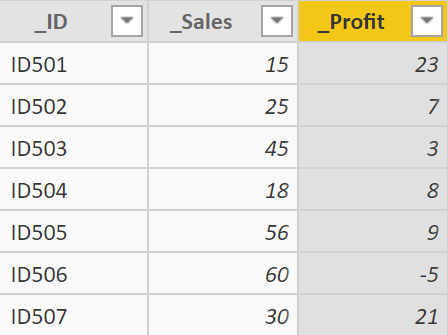#### 2.实现方法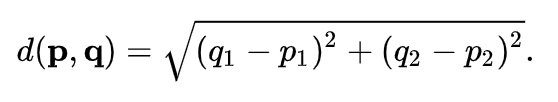``````合并集 =
VAR VT_1 =
GENERATEALL('测试集','训练集'),
"DISTANCE",
CEILING(
SQRT(('测试集'[_Sales]-'训练集'[Sales])^2+
('测试集'[_Profit]-'训练集'[Profit])^2),
0.01))
VAR VT_2 =
"IsKNN",
VAR K = 9
VAR v_id = '测试集'[_ID]
return
IF(
RANKX(
filter(VT_1,'测试集'[_ID] = v_id),
[DISTANCE],,ASC,Skip)<=K,
"T","F"))
--临近点将会被标记为"T"
VAR VT_3 =
FILTER(VT_2,[IsKNN] = "T")
RETURN VT_3
``````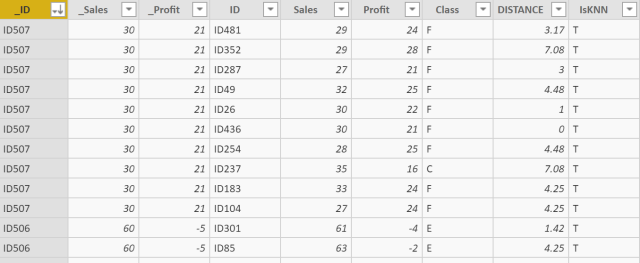``````结果集 =
VAR VT_4 =
"_Class",
VAR CLASSNUM_MAX =
CALCULATE(
MAXX('合并集',
CALCULATE(
COUNT('合并集'[Class]),
ALLEXCEPT('合并集','合并集'[_Profit],'合并集'[Class]))),
ALLEXCEPT('合并集','合并集'[_ID]))
RETURN
IF(
CLASSNUM_MAX =
CALCULATE(
COUNT('合并集'[Class]),
ALLEXCEPT('合并集','合并集'[_Profit],'合并集'[Class])),
CALCULATE(
FIRSTNONBLANK('合并集'[Class],1),
ALLEXCEPT('合并集','合并集'[_ID],'合并集'[Class])
)))
VAR VT_5 =
CALCULATETABLE(
GROUPBY(
FILTER(VT_4,[_Class]<>BLANK()),
'合并集'[_ID],
'合并集'[_Profit],
'合并集'[_Sales],
[_Class],
"Distance",
SUMX(CURRENTGROUP(),'合并集'[DISTANCE])
))
RETURN VT_5
``````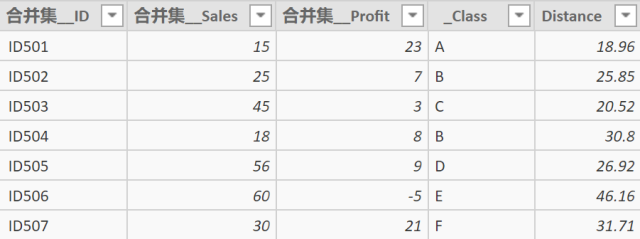``````KNN_Result =
VAR Class_ =
CALCULATE(
MIN('结果集'[Distance]),
ALLEXCEPT('结果集','结果集'[合并集__ID]))
RETURN
IF(
class_ = '结果集'[Distance],
'结果集'[_Class],
BLANK())
``````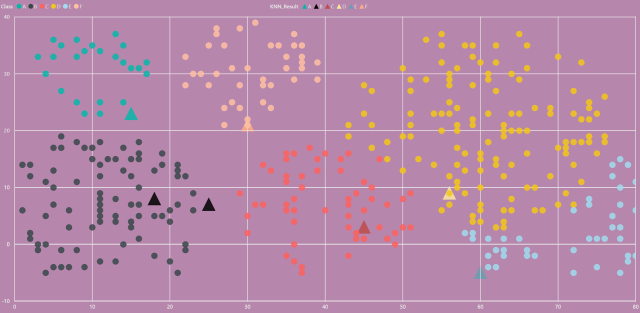## 三、其他